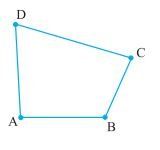Q

# Place a pair of unequal sticks such that they have their end points joined at one end. Now place another such pair meeting the free ends of the first pair. What is the figure enclosed?

Place a pair of unequal sticks such that they have their end points joined at one end. Now place another such pair meeting the             free ends of the first pair. What is the figure enclosed? It is a quadrilateral, like the one you see here. The sides of the                      quadrilateral are AB, BC , ___, ___. There are 4 angles for this quadrilateral. They are given by ∠BAD, ∠ADC, ∠DCB and _____.            BD is one diagonal. What is the other? Measure the length of the sides and the diagonals. Measure all the angles also.Views

The sides of the quadrilateral are AB, BC, CD, DA

The angles are given by